Prev: Source Code Next: Implementation Details

In here another approach to extract the view frustum planes is presented based on the properties of clip space.

Consider a point p =(x,y,z,1) on the 3D world. Consider also a modelview matrix M and a projection matrix P. The point p is transformed by matrices M and P as point pc =(xc,yc,zc,wc) in clip space using: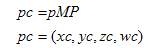The point pc is in homogeneous coordinates, and when normalised becomes pcn:In normalised clip space the view frustum is an axis aligned box centered in the origin, and bounded by the following planes:

• Left Plane: x’ = -1
• Right Plane: x’ = 1
• Top Plane: y’ = 1
• Bottom Plane: y’ = -1
• Near Plane: z’ = -1
• Far Plane: z’ = 1

This implies that the point pcn =(x’,y’,z’) is inside the view frustum if: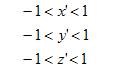Then the point pc, in non-normalized coordinates, must obbey the following conditions in order to be inside the view frustum: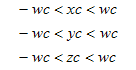Based on this information it is possible to extract the six planes, in world coordinates, that bound the view frustum. The point pc is on the “right” side of the left plane if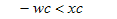Then xc and wc can be defined as a function of p = (x,y,z,w), and A.Therefore the following inequation must be true if p is on the right side of the left plane.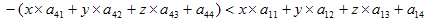So the left plane (Ax+By+Cz+D=0) is defined as:where col1 and col4 are the first and forth columns of matrix A, respectively.

If the only objective is to find out if a point is inside or outside of the frustum then the plane as defined is ok. However when testing spheres, which require computing the distance from the center of the sphere to the plane, it is advisable to normalize the plane.

The right plane can be obtained in a similar manner: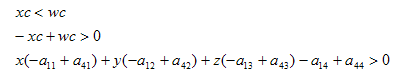The remaining planes are obtained as follows:

• Bottom Plane
•• Top Plane
•• Near Plane
•• Far Plane
•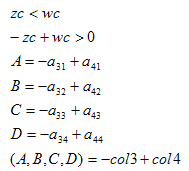Prev: Source Code Next: Implementation Details

### 4 Responses to “Clip Space Approach – Extracting the Planes”

1.I’m using OpenGL and GLM and for some reason I had to negate the plane coefficients for culling to work. I do not understand why. It took me four days and about 10 hours of trial and error and a lot of frustum drawings to figure this out. I hope it will help someone. And if anybody could explain what is going on here I would really like to hear it.

``` // Extracting the planes.```

``` mat4 matrix = projection * view; vec4 rowX = glm::row(matrix, 0); vec4 rowY = glm::row(matrix, 1); vec4 rowZ = glm::row(matrix, 2); vec4 rowW = glm::row(matrix, 3); ```

```planes = normalize(rowW + rowX); planes = normalize(rowW - rowX); planes = normalize(rowW + rowY); planes = normalize(rowW - rowY); planes = normalize(rowW + rowZ); planes = normalize(rowW - rowZ); ```

``` // Normalizing the planes.```

``` ```

```vec3 normal(plane.x, plane.y, plane.z); float length = glm::length(normal); return -plane / length; // Notice the negation. I don't know why I needed that!! ```

``` // Sphere intersection test.```

``` ```

```for (vec4 plane : planes) { float dist = plane.x * center.x + plane.y * center.y + plane.z * center.z + plane.w - radius; if (dist > 0) return false; } return true; ```

2.The extracting from the planes is time consuming and the
testing later problematic in my opinion.
If have found another solution.
My destination was, to found a good way to make frustum
clipping with a bounding sphere.
Based on the fact, that the objects center lies already in a
modelviewprojection matrix, i use it for my culling.
Here the method (in java):

public boolean isSphereInFrustum( double fieldOfView, double nearPlaneDistance, double aspectRatio, Vector4d center, double radius )
{
// the center from the bounding sphere, taken from the
// modelviewprojection matrix
double px = center.x;
double py = center.y;
double pz = center.z;
// make positive
if ( pz<0.0)
pz = -pz;
// scaling to screen
double scale = nearPlaneDistance/(nearPlaneDistance+pz);
// make positive
if ( px<0.0)
px = -px;
// the sphere-width on screen
if ( py<0.0)
py = -py;
// the sphere-height on screen
// test for objectsize on screen, goes out if sphere only a pixel
if ( tmpX<1.0 && tmpYx || py>y || pz>80 || pz<0.1 )
return false;
return true;
}

3.Hi

I use the opengl column-major matrix layout :
a00 a10 a20 a30
a01 a11 a21 a31
a02 a12 a22 a32
a03 a13 a23 a33
————————
col1 col2 col3 col4
Now, if i take the planes from my modelViewProjection-Matrix
i got for the left plane = col1+col4 a plane that lies in the xz-plane.
must the left plane not be in the yz-plane?

•Hi Marvin,

The left plane in clip space is parallel to the yz plane.

This site uses Akismet to reduce spam. Learn how your comment data is processed.